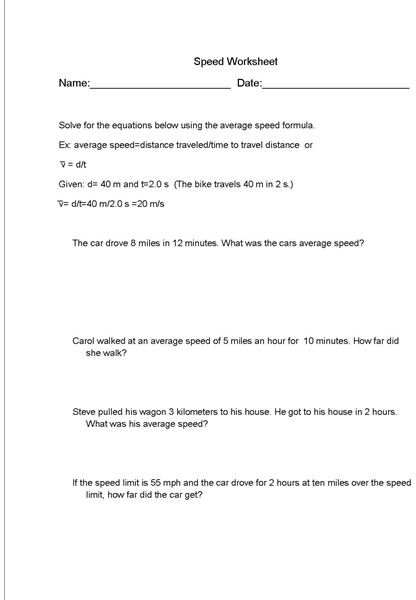Speed Lesson Plans for Science

Teaching Students About Speed

Teachers use this lesson to develop student’s understanding of the speed formula. Before completing this speed formula lesson plan, students should be familiar with the concept of motion and what it entails. Review the following information on speed as it applies to motion with your students. Read the following information to your students, then do the board work listed below.

Speed is a scalar quantity. It has only magnitude and no direction. Speed is a basic description of motion. In other words, it is a way to measure motion. An average speed can be determined if the length and time of the motion are known. Likewise the length of motion can be known if the average speed and the time of the motion are known. The time of the motion can also be found by using the speed formula if the length of the motion and the actual speed are known.

Speed Formula Board Work

Write the following problems on the board and then ask students to decide what further information, if any is required to complete the problem.

1. A car traveled for 3 miles. How long did it travel?
2. The marble rolled with an average speed of 2 m/s. How far did the marble go?
3. Tom rode his bike at 2 miles an hour for 12 miles. How long did it take him to finish his ride?
4. Sam walked for 5 miles. What was Steve’s average speed?
5. If a log rolled for 10 minutes and went 1 mile, what was the average speed of the log?

At the end of the board work exercise, students should be aware of what quantities are required in order to use the speed formula to get the answer to the problems. Students should not be able to complete the speed formula worksheet that is included in this lesson plan. If students require further assistance in understanding speed and how to use the speed formula, direct them to this speed formula study guide that goes with this speed lesson plan.

Speed Formula WorksheetReferences

• Student teaching experience.

This post is part of the series: Motion in Physical Science

This series on motion in physical science comes complete with worksheets and study guides for motion, speed, velocity and acceleration.